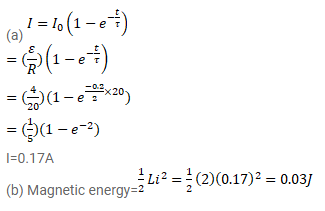# A coil having inductance 2.0 H and resistance 20Ω

Question:

A coil having inductance $2.0 \mathrm{H}$ and resistance $20 \Omega$ is connected to a battery of emf $4.0 \mathrm{~V}$. Find (a) the current at the instant $0.20$ s after the connection is made and (b) the magnetic field energy at this instant.

Solution: